# NCERT Solutions for Class 9 Science Chapter 4- Structure Of The Atom

## NCERT Solutions for Class 9 Science Chapter 4 - CBSE Term II Free PDF Download

NCERT Solutions for Class 9 Science Chapter 4 Structure of The Atom at BYJU'S provides students with solutions to all the questions provided in the NCERT Class 9 textbook, which is on the lines of the CBSE board. This article provides students with the study source material that is crafted by a team of experts with in-depth knowledge of the subject. Solving NCERT Solutions for Class 9 Science would enable students to score maximum marks in the Class 9 CBSE  Science Term II exam.

The language used while preparing the NCERT Solutions for Class 9 Science by our experts at BYJU'S is proficient and to the understanding of the Class 9 students, thereby assisting them in understanding the concept better. This is established on the concept-based approach. These NCERT Solutions are curated keeping in mind the answering method that is promoted by the CBSE and hence the allotment of marks in CBSE Term II examinations is taken into consideration for students to lay out their answers correspondingly and hence to score maximum marks. The NCERT textbook provided by the board is designed by the panel taking into consideration the latest in the field of science and technology.

### Download PDF of NCERT Solutions for Class 9 Science Chapter 4 - Structure Of The Atom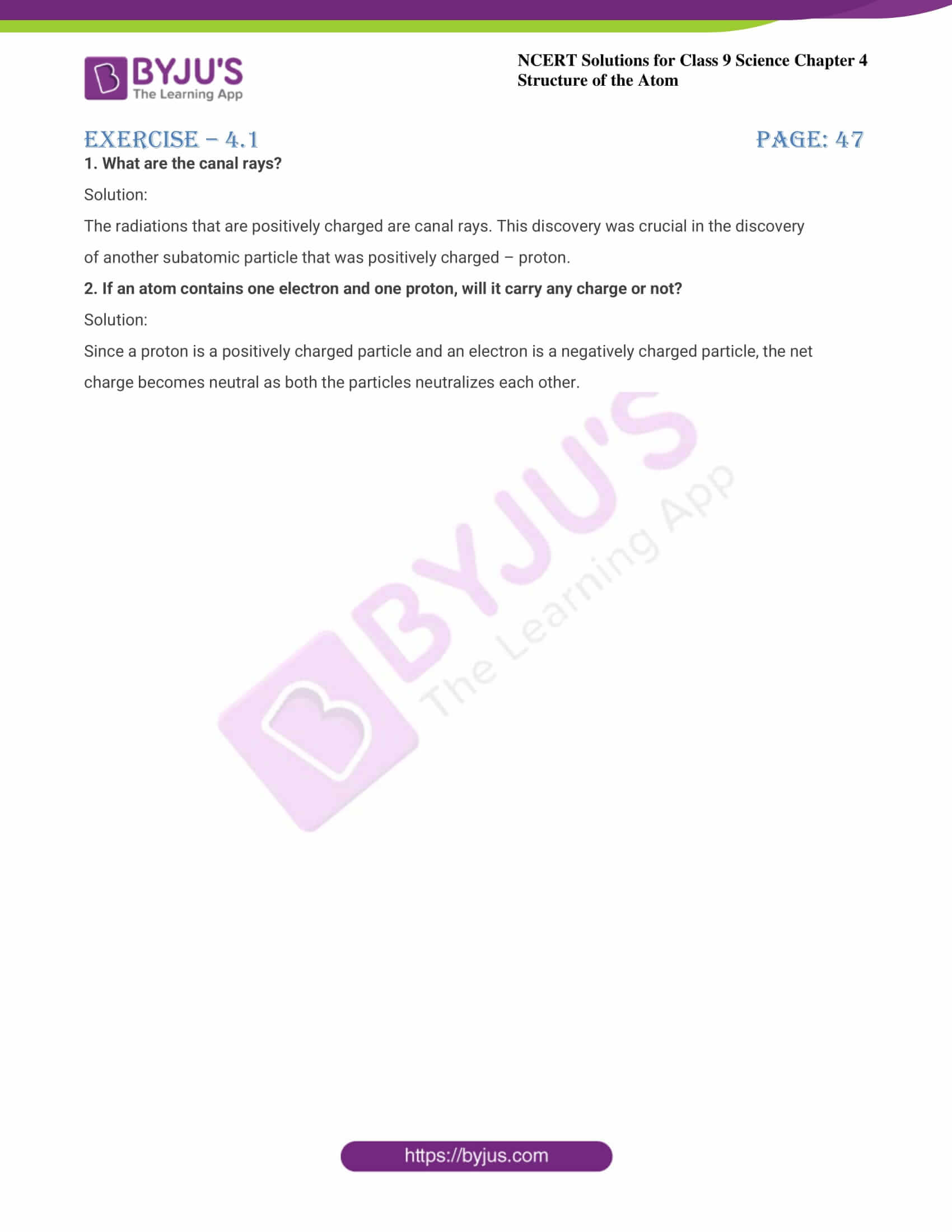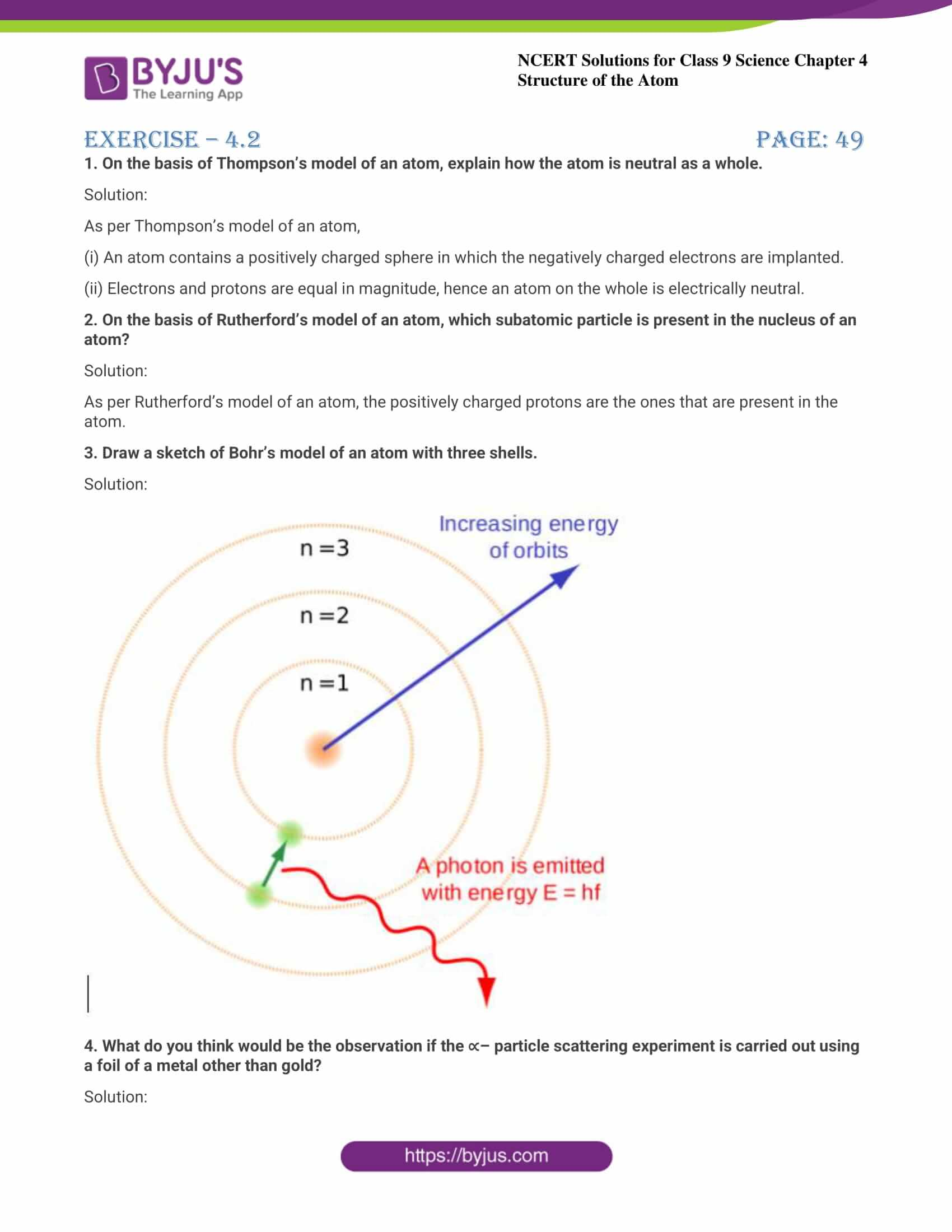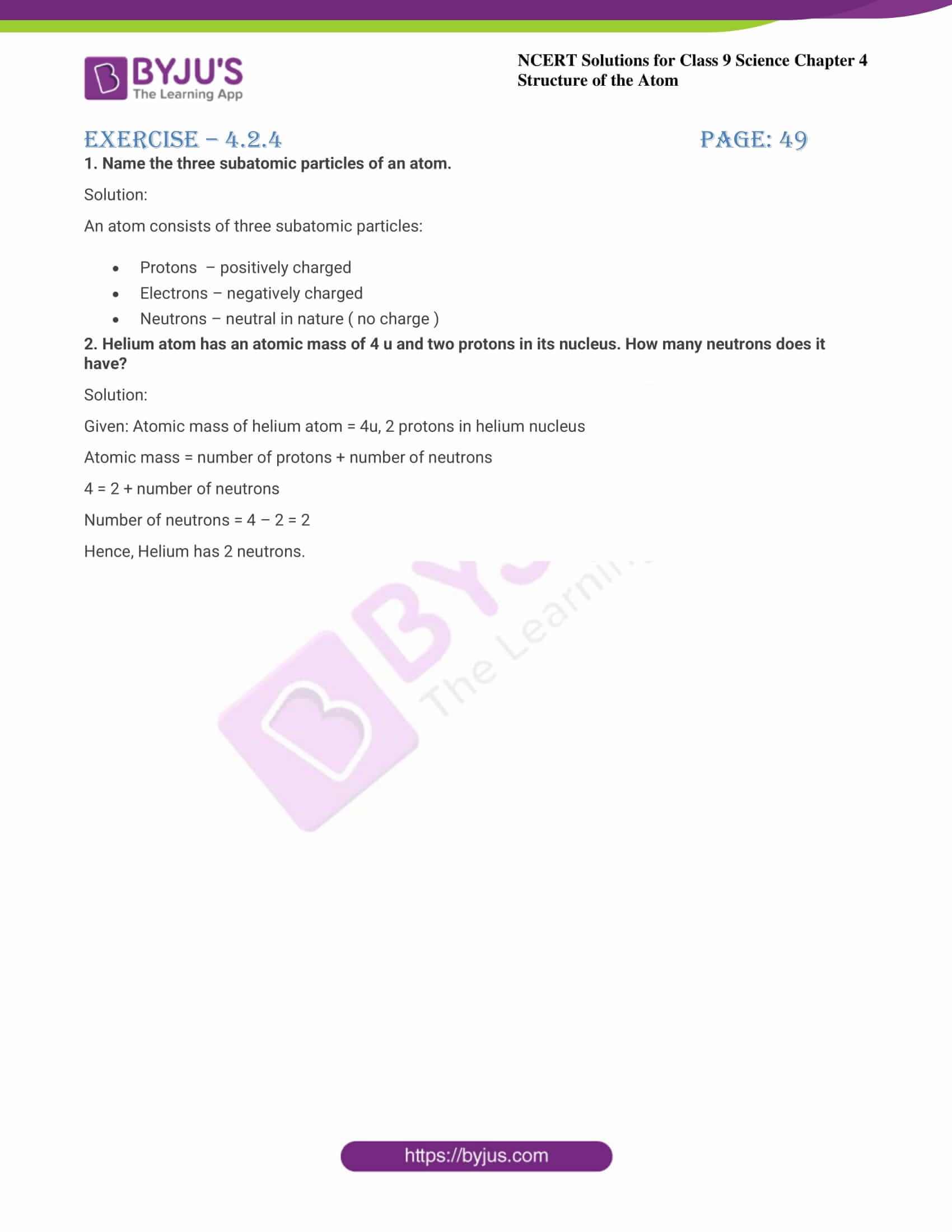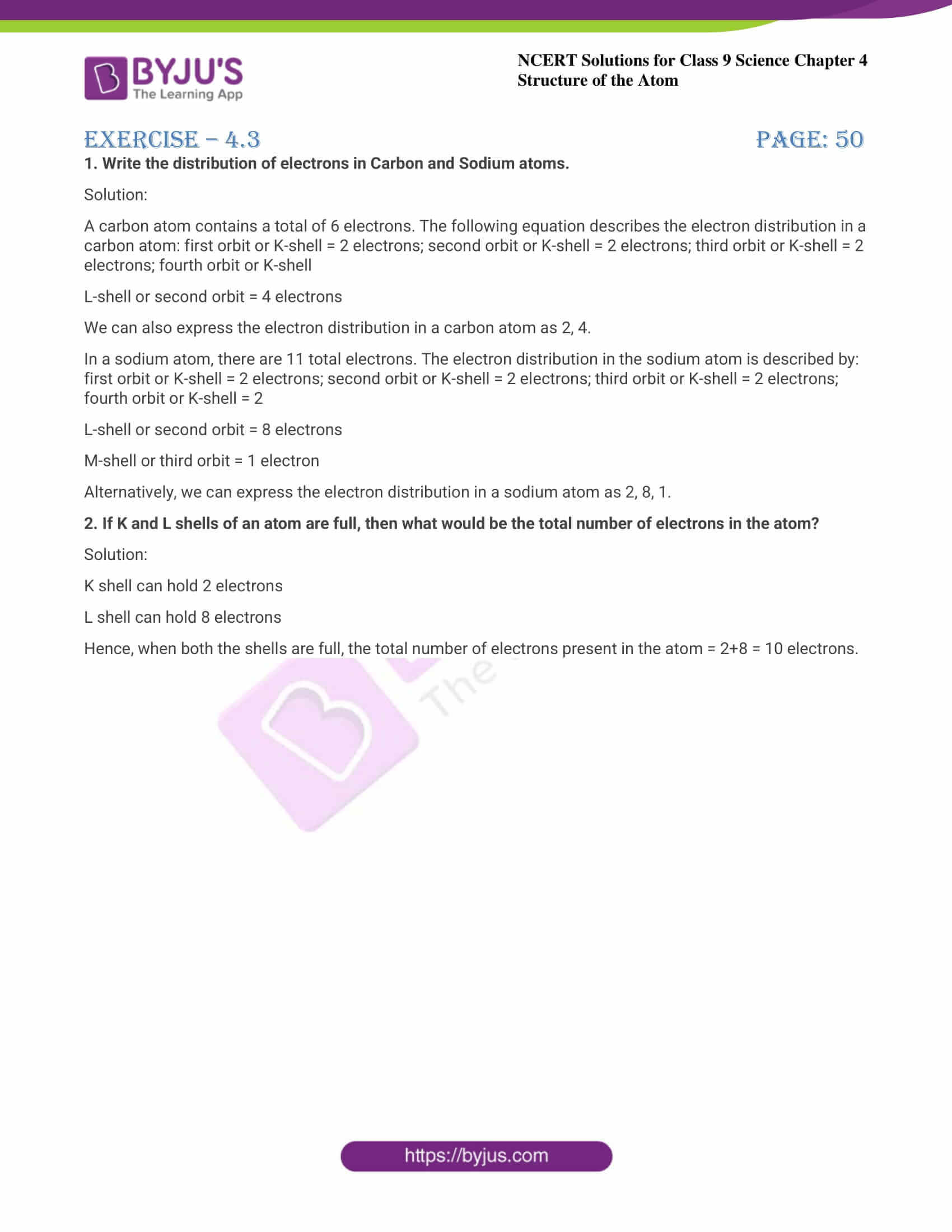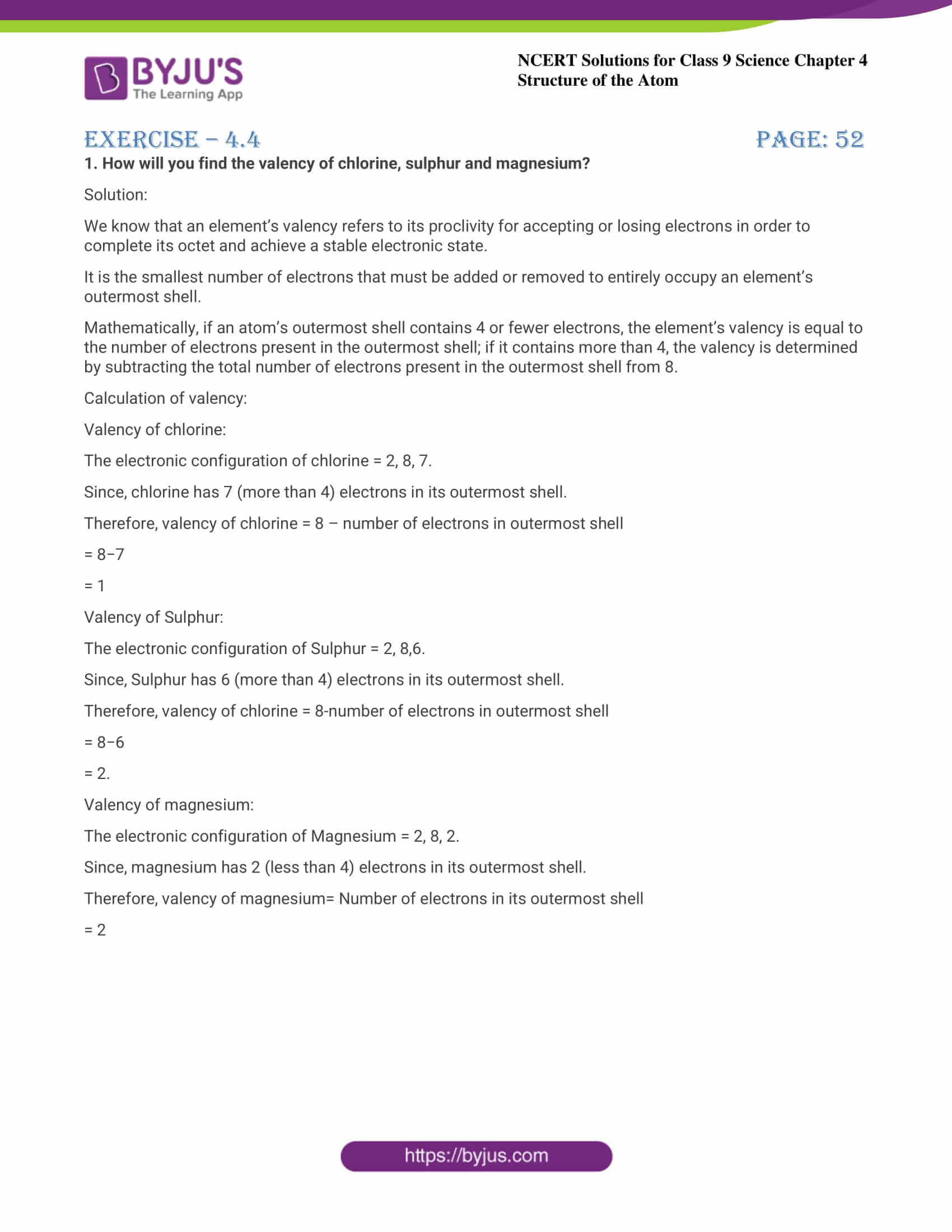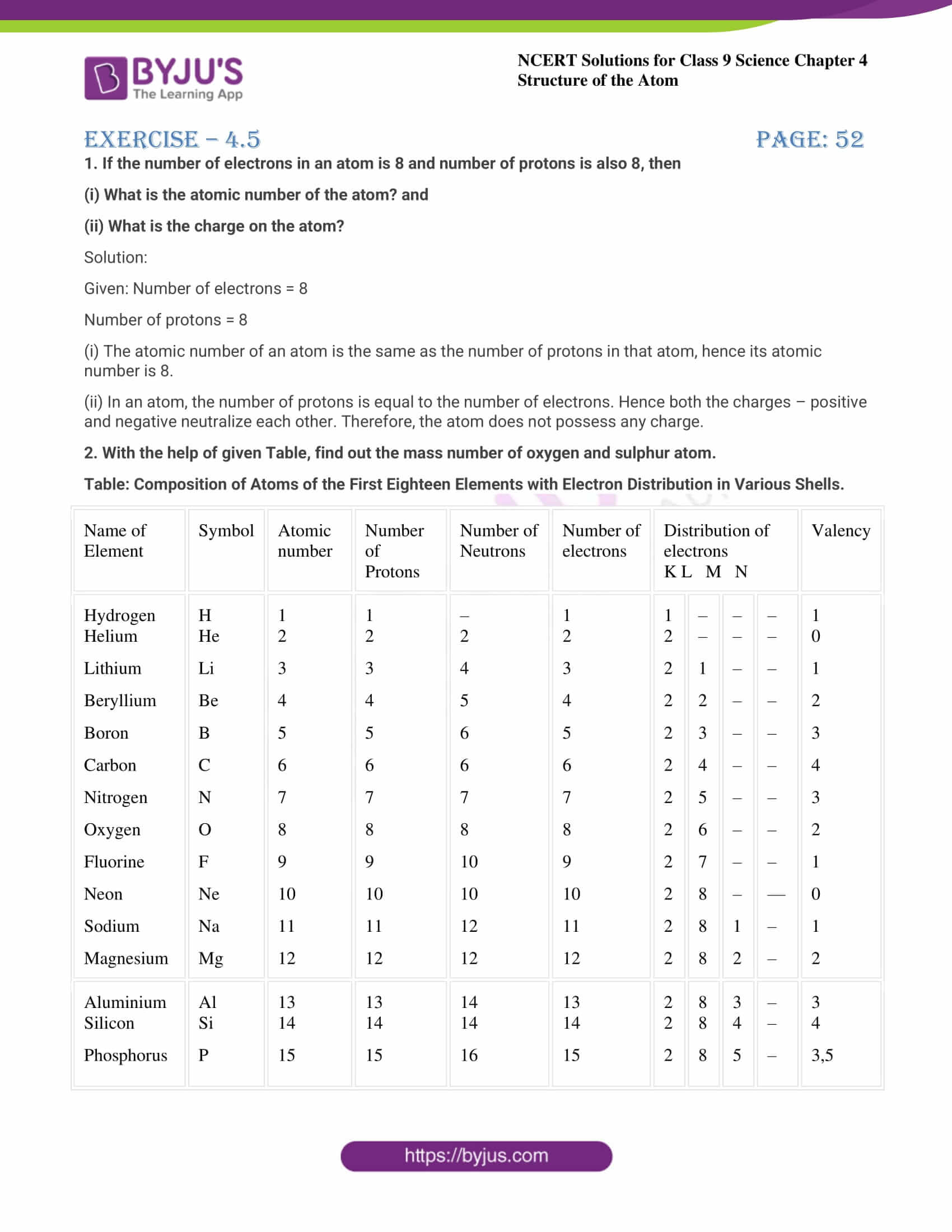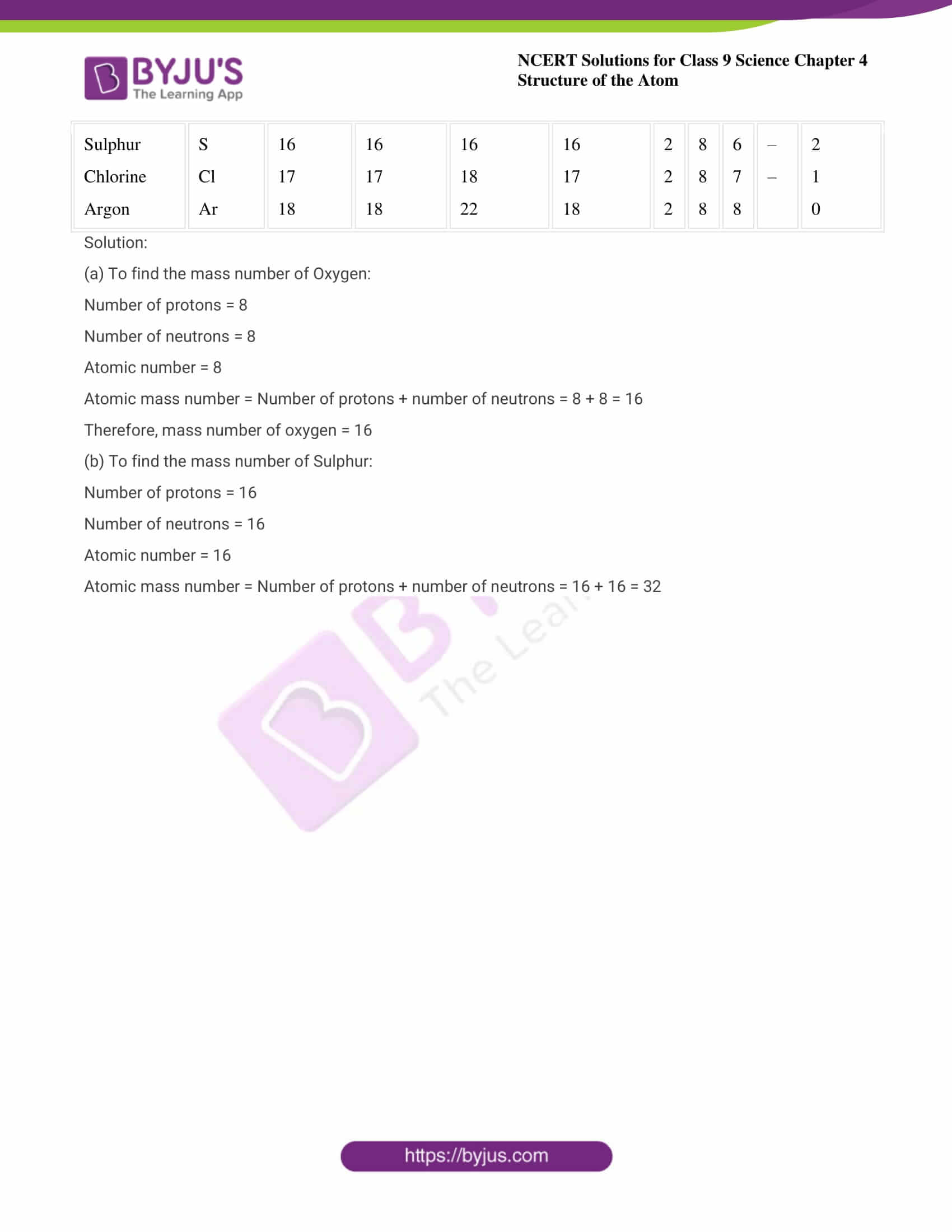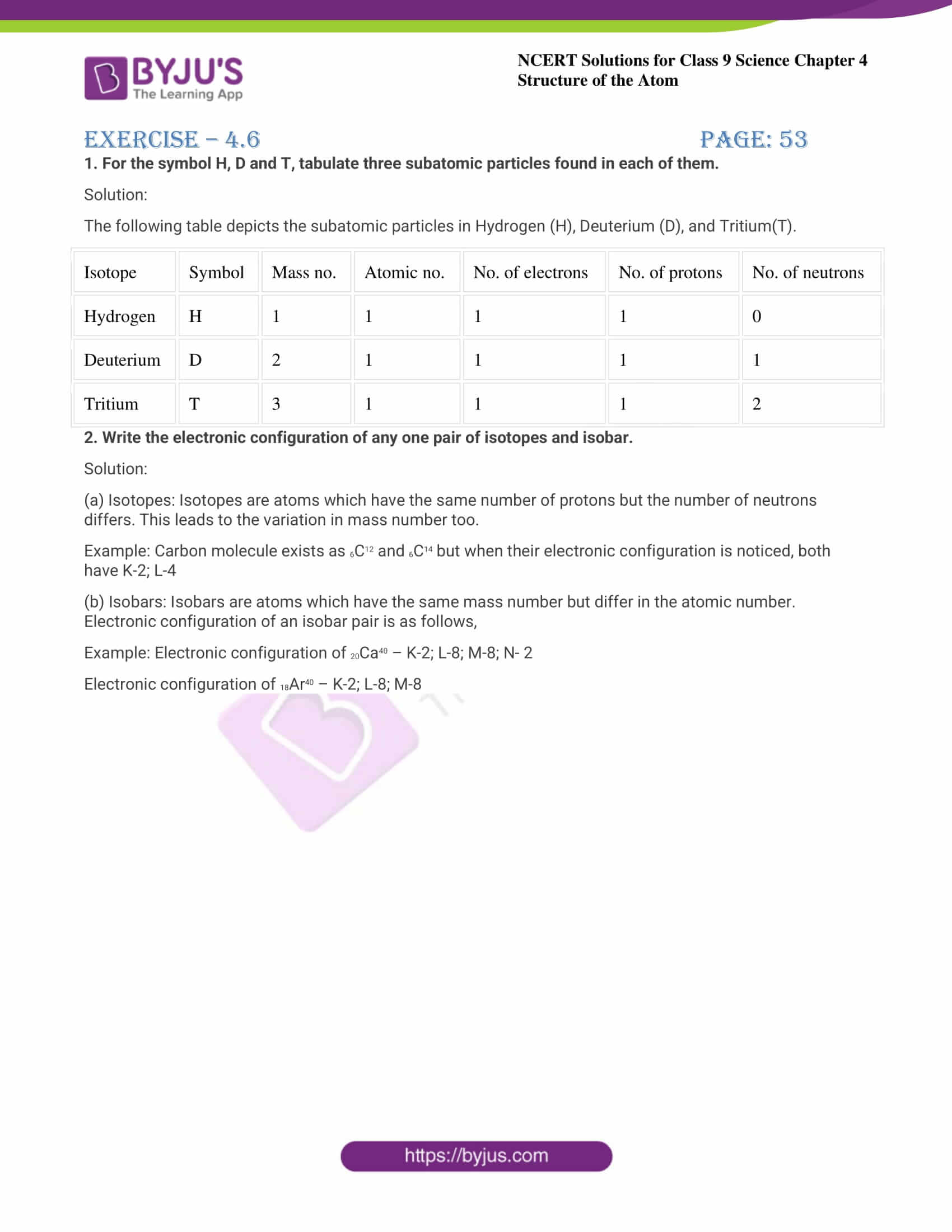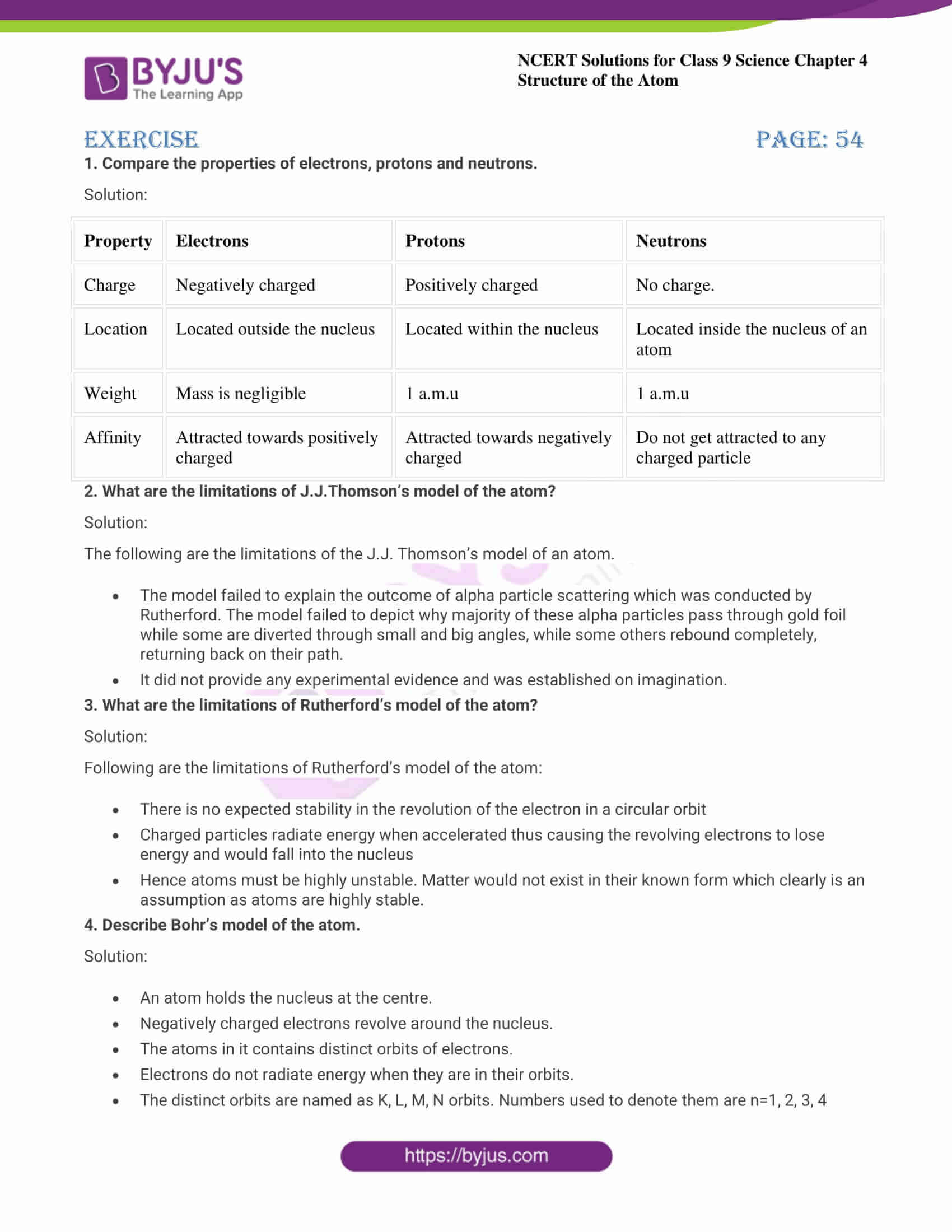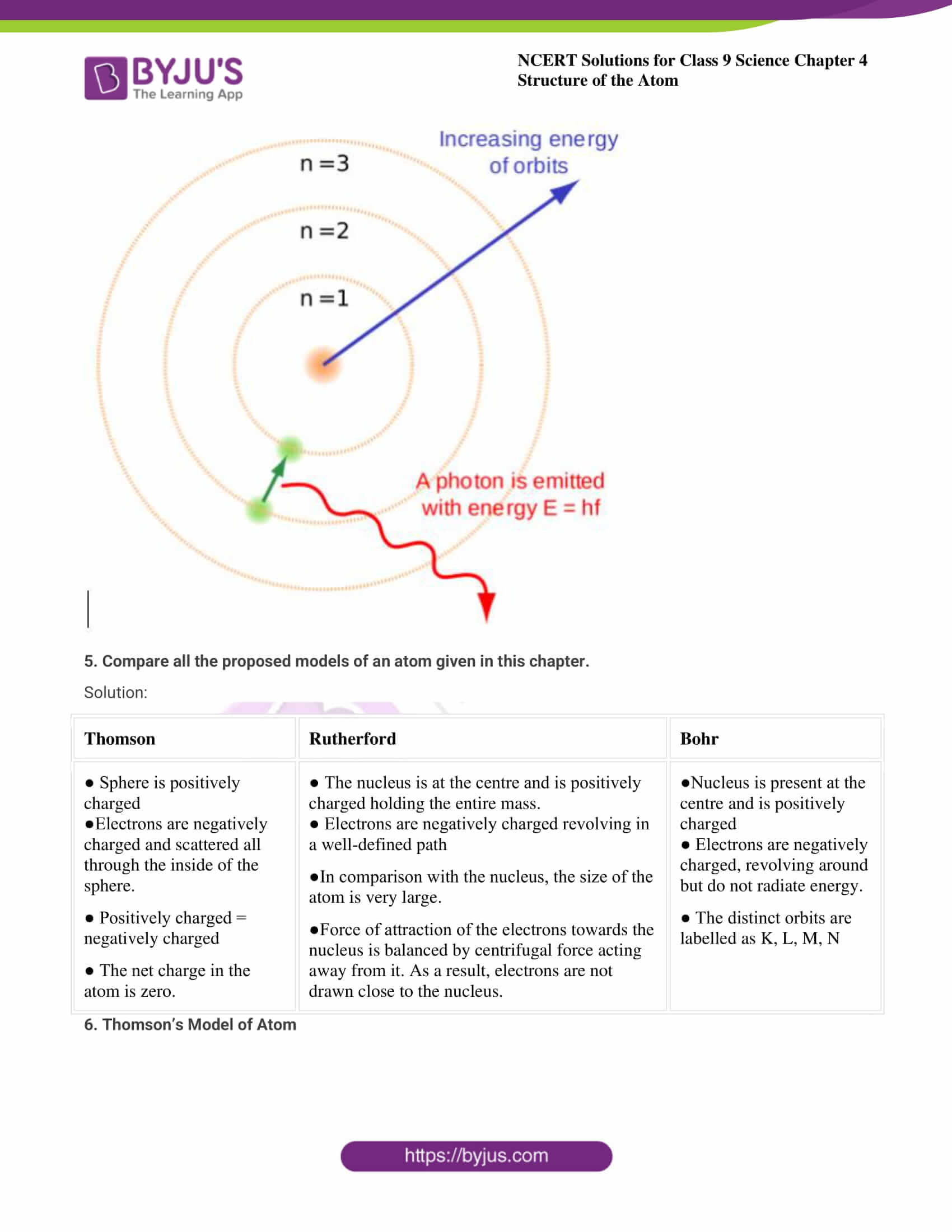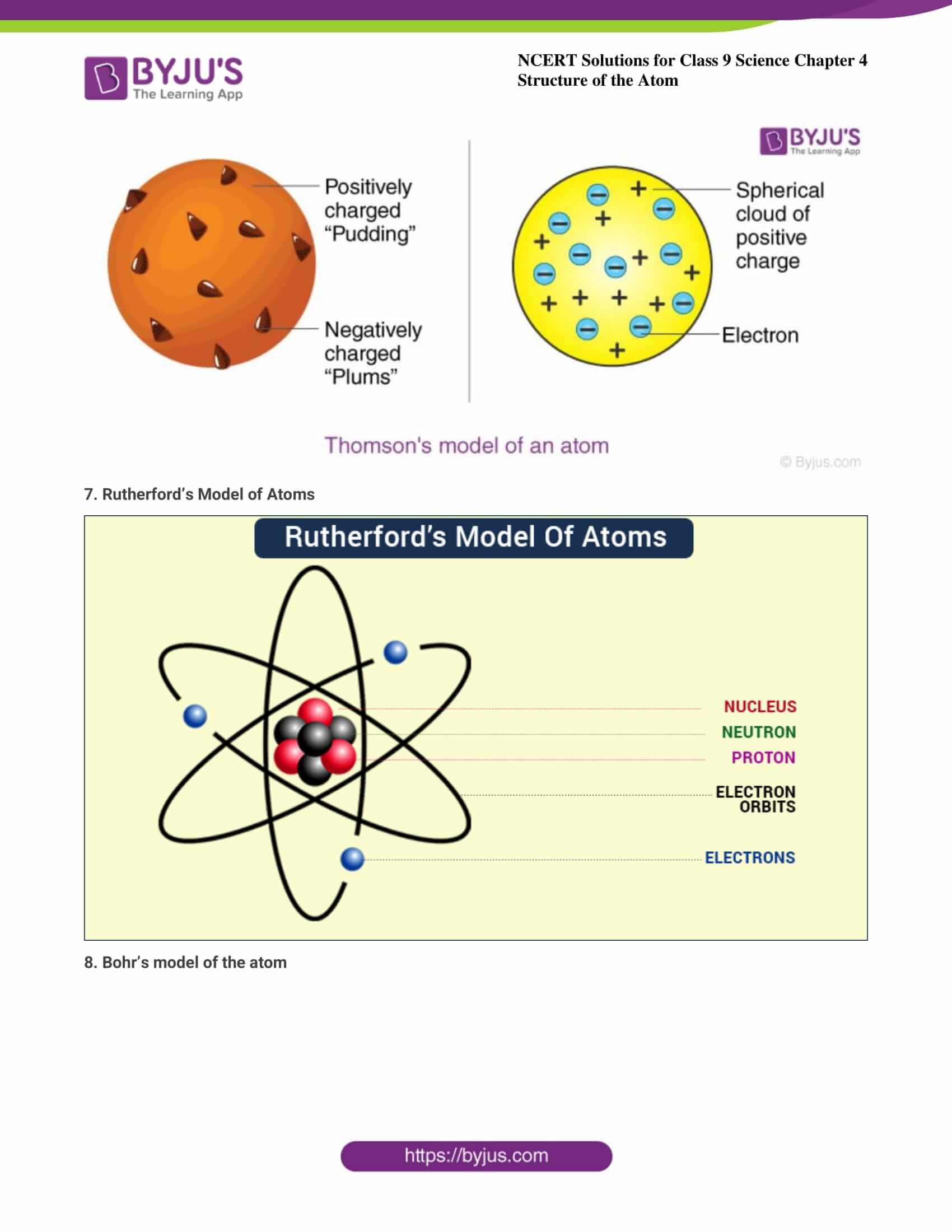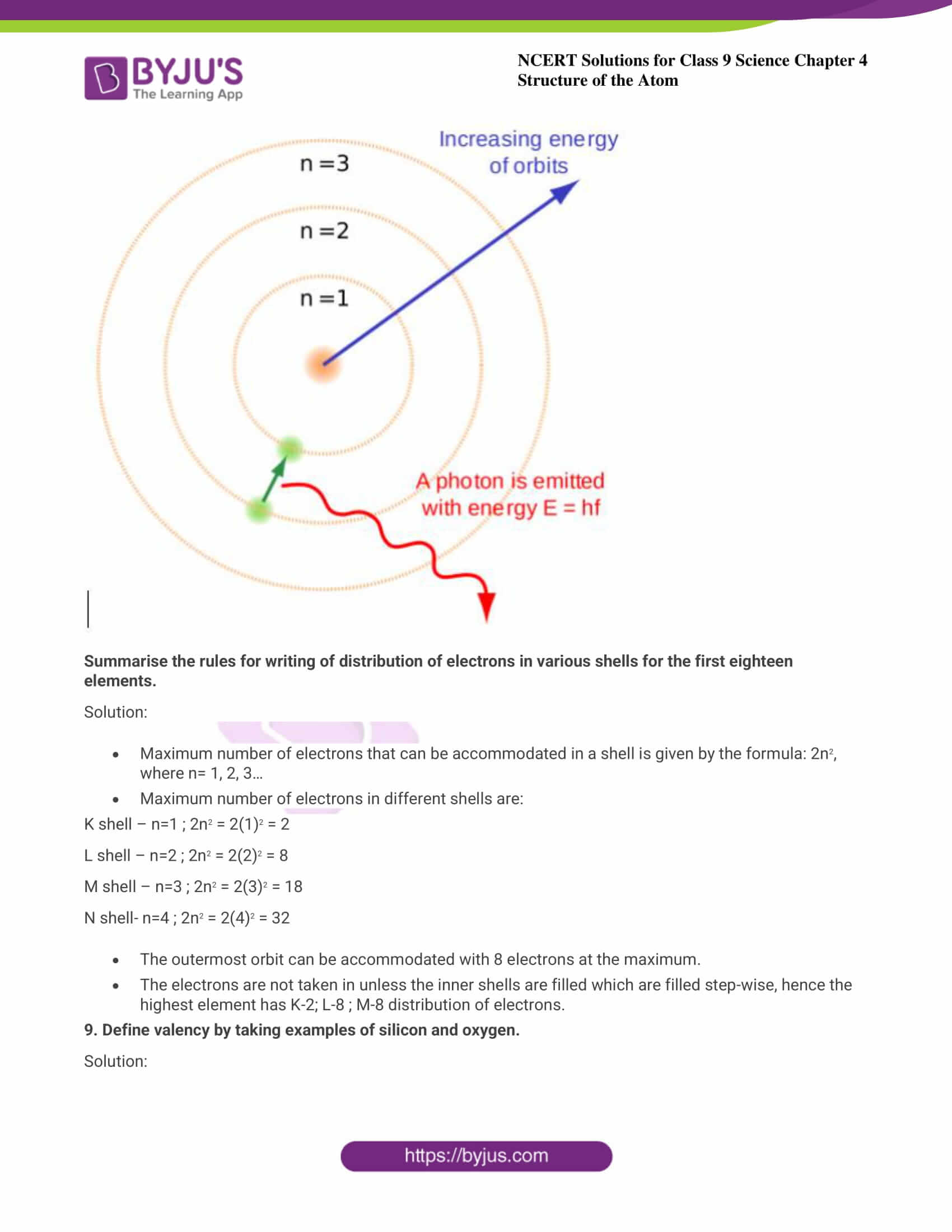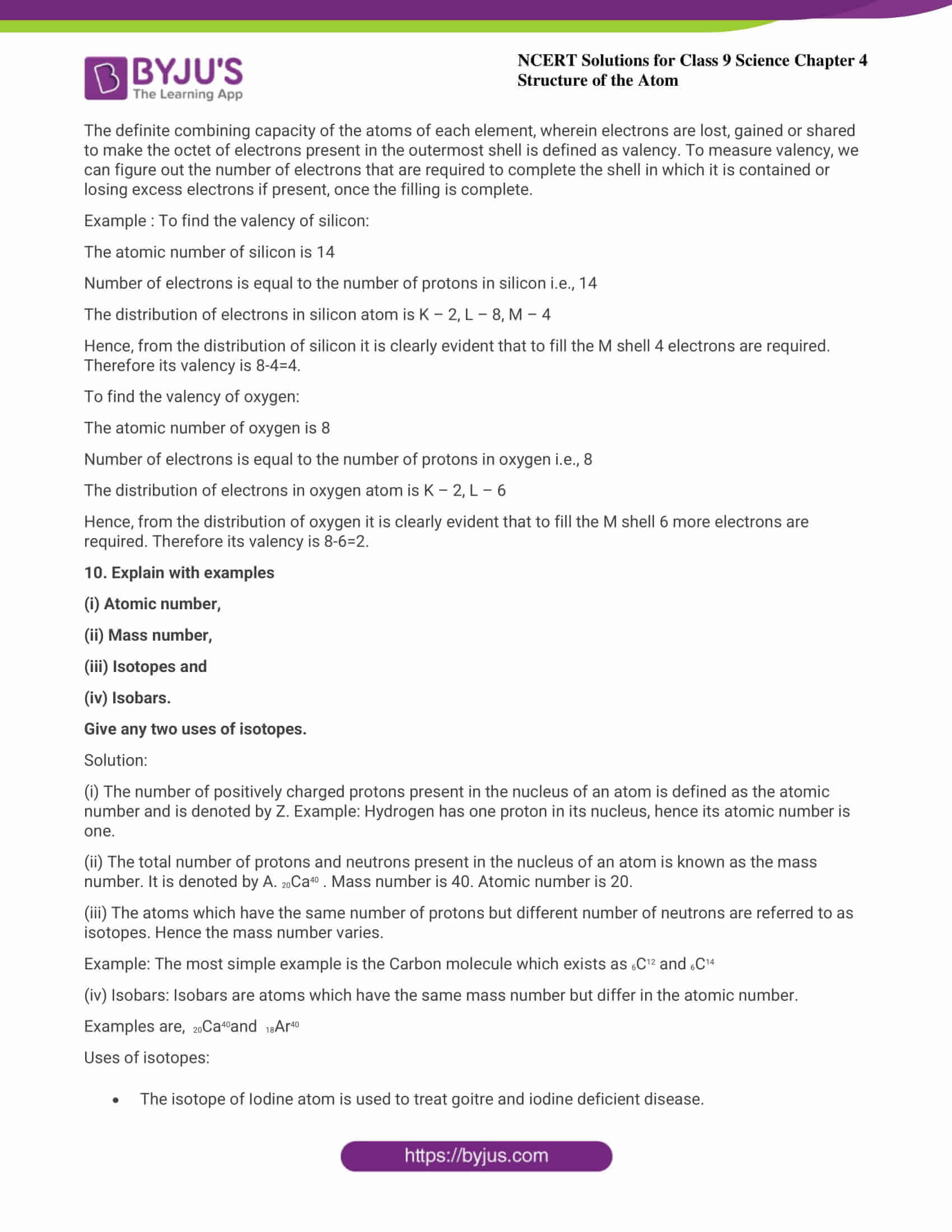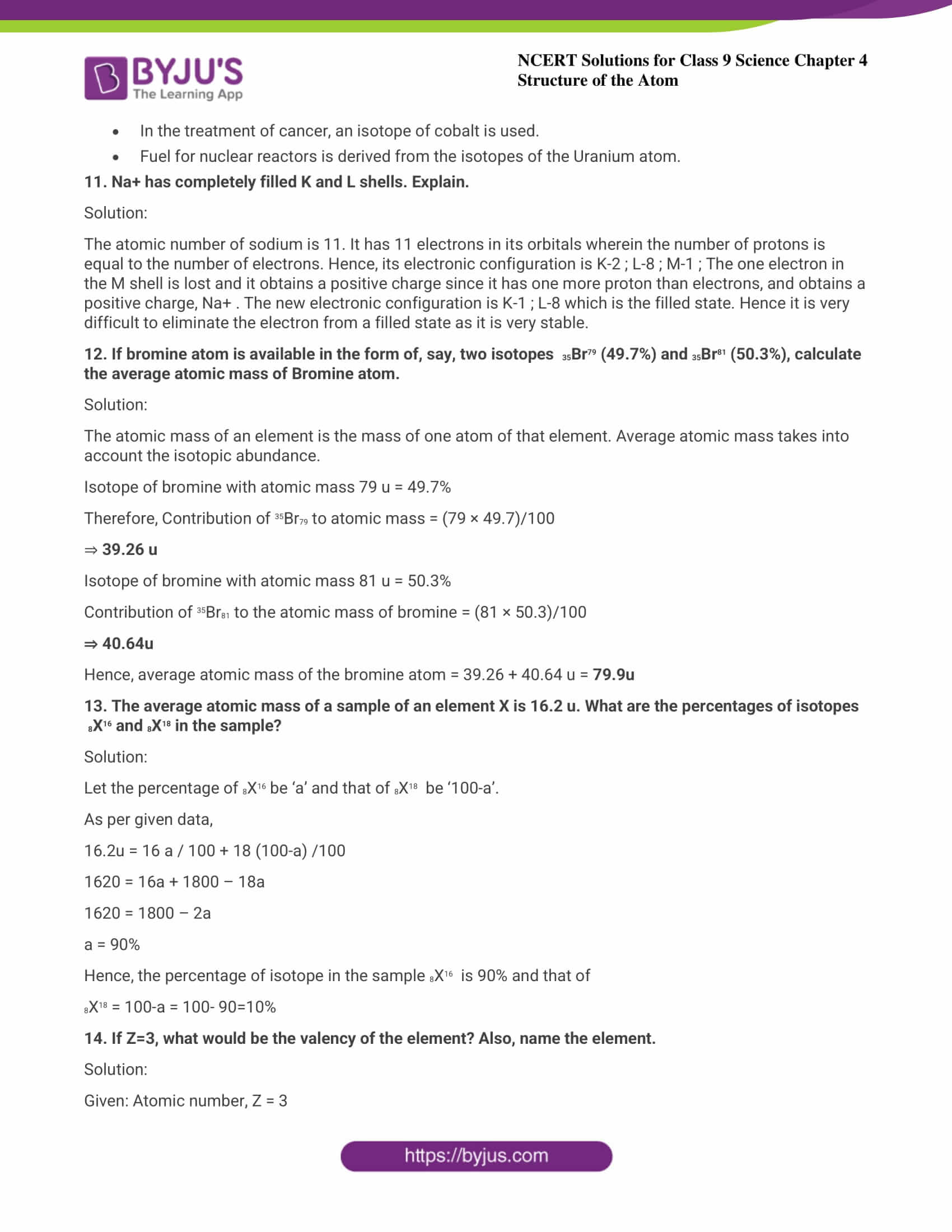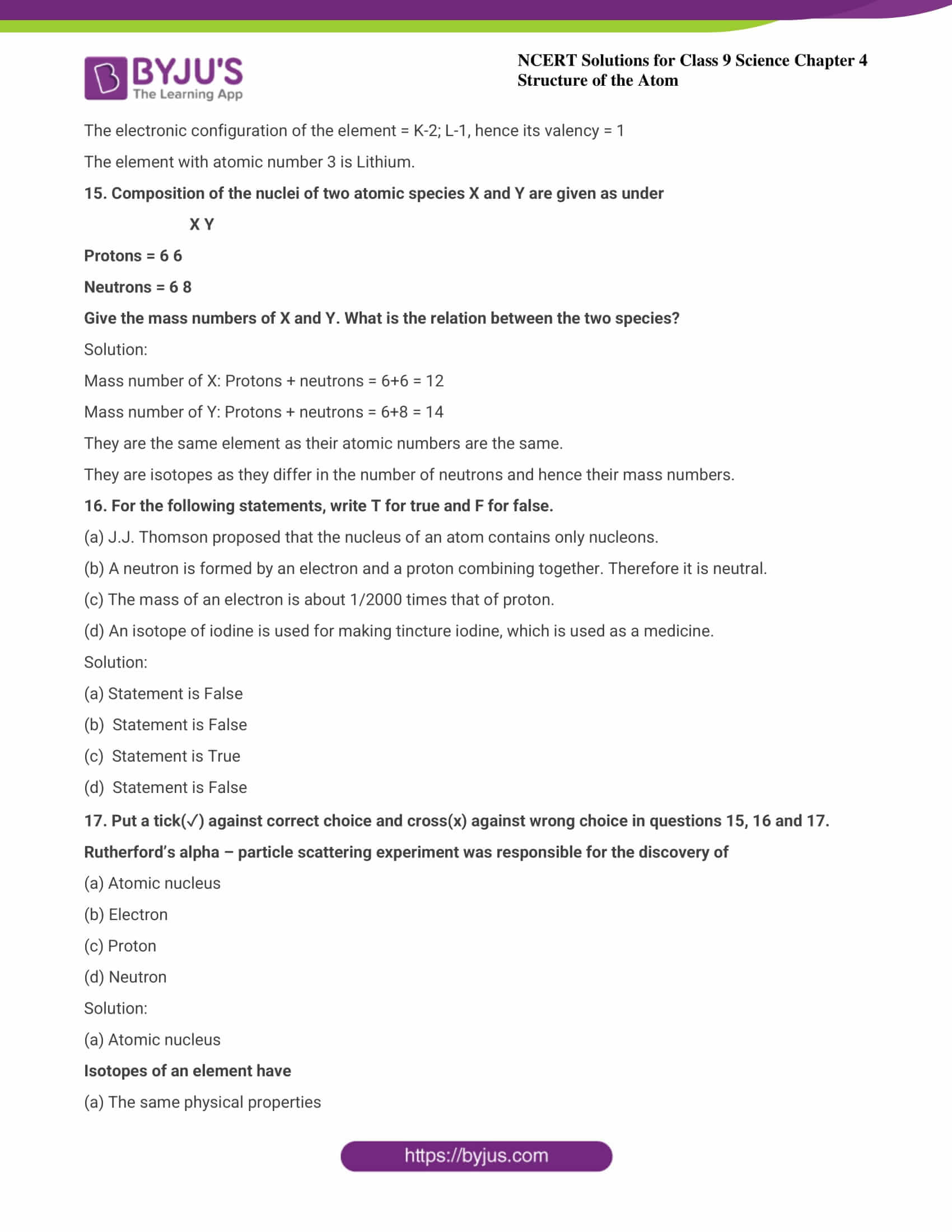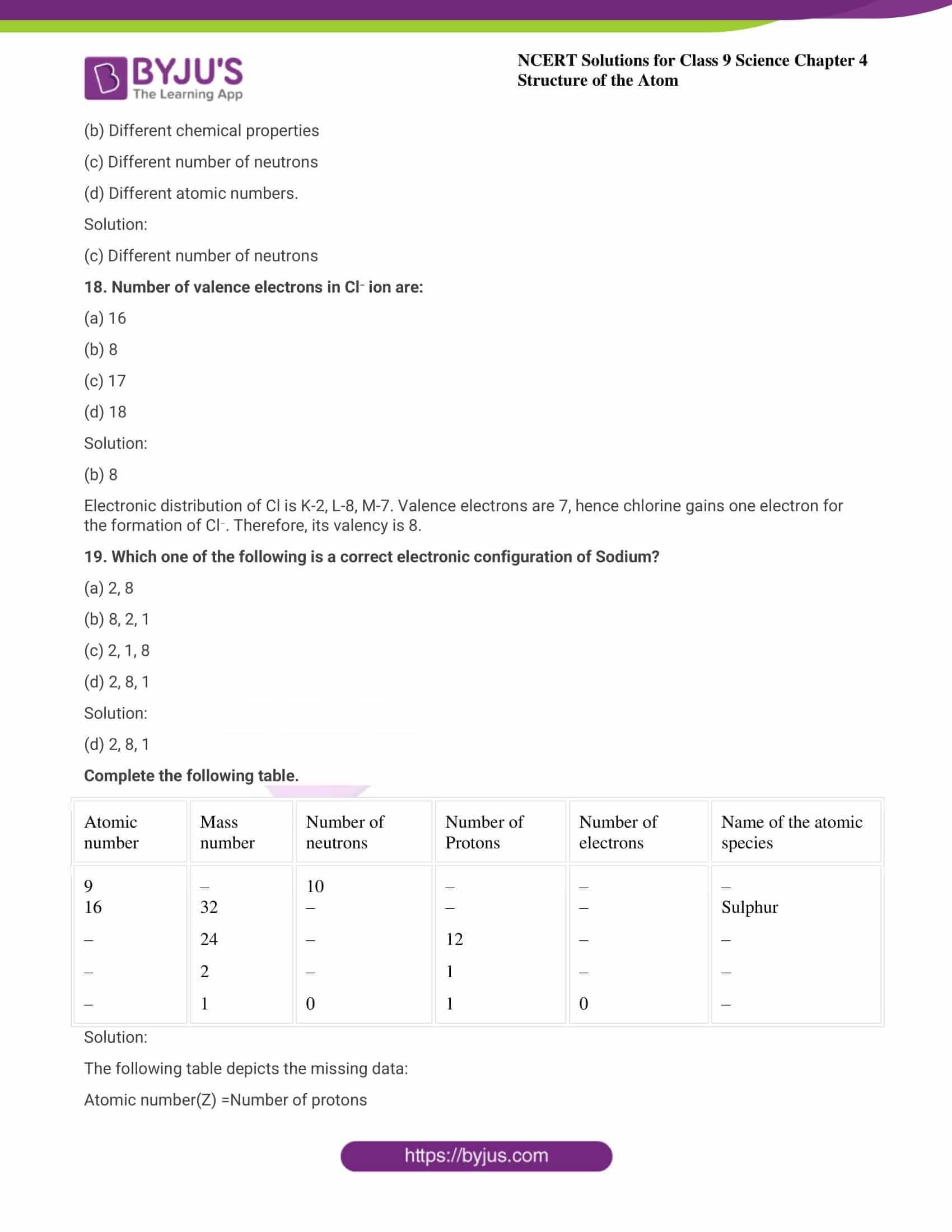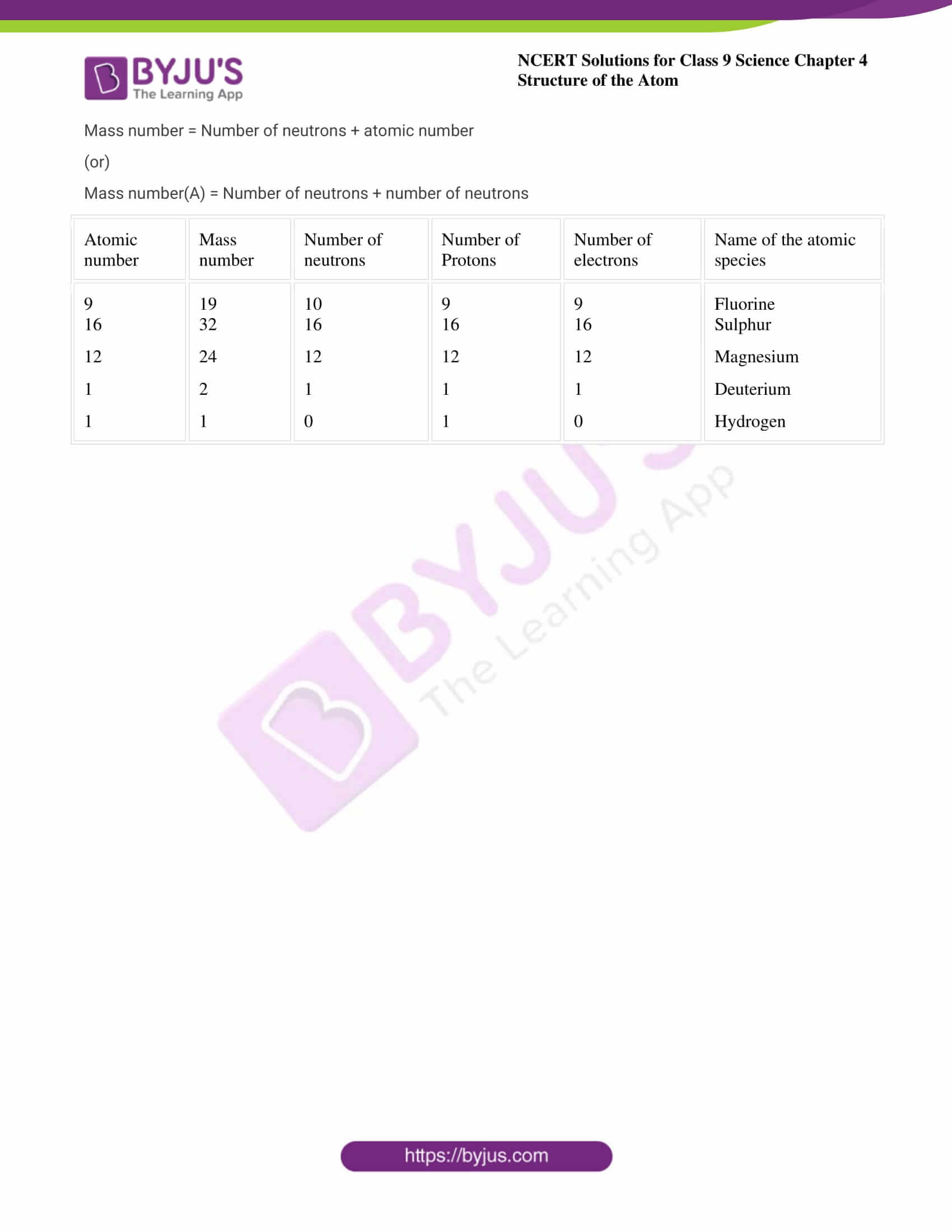## Exercise-4.1 Page: 47

1. What are the canal rays? Solution: The radiations that are positively charged are canal rays. This discovery was crucial in the discovery of another subatomic particle that was positively charged - proton. 2. If an atom contains one electron and one proton, will it carry any charge or not? Solution: Since a proton is a positively charged particle and an electron is a negatively charged particle, the net charge becomes neutral as both the particles neutralizes each other.

## Exercise-4.2 Page: 49

1. On the basis of Thompson’s model of an atom, explain how the atom is neutral as a whole. Solution: As per Thompson’s model of an atom, (i) An atom contains a positively charged sphere in which the negatively charged electrons are implanted. (ii) Electrons and protons are equal in magnitude, hence an atom on the whole is electrically neutral. 2. On the basis of Rutherford’s model of an atom, which subatomic particle is present in the nucleus of an atom? Solution: As per Rutherford’s model of an atom, the positively charged protons are the ones that are present in the atom. 3. Draw a sketch of Bohr’s model of an atom with three shells. Solution: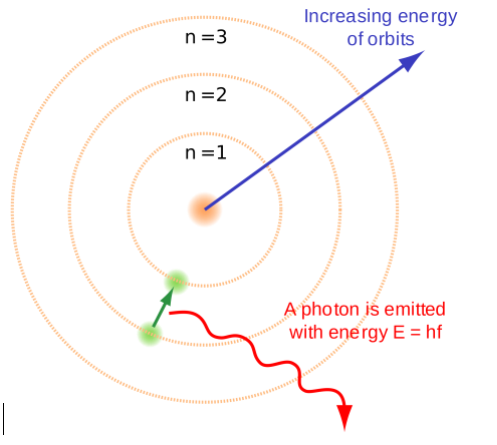4. What do you think would be the observation if the ∝– particle scattering experiment is carried out using a foil of a metal other than gold? Solution: In the ∝ – particle scattering experiment, when any other metal foil is used instead of gold, the observation would remain the same. This is because the structure of an atom when considered individually remains the same.

## Exercise-4.2.4 Page: 49

1. Name the three subatomic particles of an atom. Solution: An atom consists of three subatomic particles:
• Protons  - positively charged
• Electrons - negatively charged
• Neutrons - neutral in nature ( no charge )
2. Helium atom has an atomic mass of 4 u and two protons in its nucleus. How many neutrons does it have? Solution: Given: Atomic mass of helium atom = 4u, 2 protons in helium nucleus Atomic mass = number of protons + number of neutrons 4 = 2 + number of neutrons Number of neutrons = 4 – 2 = 2 Hence, Helium has 2 neutrons.

## Exercise-4.3 Page: 50

1. Write the distribution of electrons in Carbon and Sodium atoms. Solution: A carbon atom contains a total of 6 electrons. The following equation describes the electron distribution in a carbon atom: first orbit or K-shell = 2 electrons; second orbit or K-shell = 2 electrons; third orbit or K-shell = 2 electrons; fourth orbit or K-shell L-shell or second orbit = 4 electrons We can also express the electron distribution in a carbon atom as 2, 4. In a sodium atom, there are 11 total electrons. The electron distribution in the sodium atom is described by: first orbit or K-shell = 2 electrons; second orbit or K-shell = 2 electrons; third orbit or K-shell = 2 electrons; fourth orbit or K-shell = 2 L-shell or second orbit = 8 electrons M-shell or third orbit = 1 electron Alternatively, we can express the electron distribution in a sodium atom as 2, 8, 1. 2. If K and L shells of an atom are full, then what would be the total number of electrons in the atom? Solution: K shell can hold 2 electrons L shell can hold 8 electrons Hence, when both the shells are full, the total number of electrons present in the atom = 2+8 = 10 electrons.

## Exercise-4.4 Page: 52

1. How will you find the valency of chlorine, sulphur and magnesium? Solution: We know that an element's valency refers to its proclivity for accepting or losing electrons in order to complete its octet and achieve a stable electronic state. It is the smallest number of electrons that must be added or removed to entirely occupy an element's outermost shell. Mathematically, if an atom's outermost shell contains 4 or fewer electrons, the element's valency is equal to the number of electrons present in the outermost shell; if it contains more than 4, the valency is determined by subtracting the total number of electrons present in the outermost shell from 8. Calculation of valency: Valency of chlorine:  The electronic configuration of chlorine = 2, 8, 7. Since, chlorine has 7 (more than 4) electrons in its outermost shell. Therefore, valency of chlorine = 8 - number of electrons in outermost shell = 8−7 = 1 Valency of Sulphur:  The electronic configuration of Sulphur = 2, 8,6. Since, Sulphur has 6 (more than 4) electrons in its outermost shell. Therefore, valency of chlorine = 8-number of electrons in outermost shell = 8−6 = 2. Valency of magnesium:  The electronic configuration of Magnesium = 2, 8, 2. Since, magnesium has 2 (less than 4) electrons in its outermost shell. Therefore, valency of magnesium= Number of electrons in its outermost shell = 2

## Exercise-4.5 Page: 52

1. If the number of electrons in an atom is 8 and number of protons is also 8, then (i) What is the atomic number of the atom? and (ii) What is the charge on the atom? Solution: Given: Number of electrons = 8 Number of protons = 8 (i) The atomic number of an atom is the same as the number of protons in that atom, hence its atomic number is 8. (ii) In an atom, the number of protons is equal to the number of electrons. Hence both the charges - positive and negative neutralize each other. Therefore, the atom does not possess any charge. 2. With the help of given Table, find out the mass number of oxygen and sulphur atom. Table: Composition of Atoms of the First Eighteen Elements with Electron Distribution in Various Shells.
 Name of Element Symbol Atomic number Number of Protons Number of Neutrons Number of electrons Distribution of electrons K L   M   N Valency Hydrogen Helium Lithium Beryllium Boron Carbon Nitrogen Oxygen Fluorine Neon Sodium Magnesium H He Li Be B C N O F Ne Na Mg 1 2 3 4 5 6 7 8 9 10 11 12 1 2 3 4 5 6 7 8 9 10 11 12 – 2 4 5 6 6 7 8 10 10 12 12 1 2 3 4 5 6 7 8 9 10 11 12 1 2 2 2 2 2 2 2 2 2 2 2 – – 1 2 3 4 5 6 7 8 8 8 – – – – – – – – – – 1 2 – – – – – – – – – — – – 1 0 1 2 3 4 3 2 1 0 1 2 Aluminium Silicon Phosphorus Sulphur Chlorine Argon Al Si P S Cl Ar 13 14 15 16 17 18 13 14 15 16 17 18 14 14 16 16 18 22 13 14 15 16 17 18 2 2 2 2 2 2 8 8 8 8 8 8 3 4 5 6 7 8 – – – – – 3 4 3,5 2 1 0
Solution: (a) To find the mass number of Oxygen: Number of protons = 8 Number of neutrons = 8 Atomic number = 8 Atomic mass number = Number of protons + number of neutrons = 8 + 8 = 16 Therefore, mass number of oxygen = 16 (b) To find the mass number of Sulphur: Number of protons = 16 Number of neutrons = 16 Atomic number = 16 Atomic mass number = Number of protons + number of neutrons = 16 + 16 = 32

## Exercise-4.6 Page: 53

1. For the symbol H, D and T, tabulate three subatomic particles found in each of them. Solution: The following table depicts the subatomic particles in Hydrogen (H), Deuterium (D), and Tritium(T).
 Isotope Symbol Mass no. Atomic no. No. of electrons No. of protons No. of neutrons Hydrogen H 1 1 1 1 0 Deuterium D 2 1 1 1 1 Tritium T 3 1 1 1 2
2. Write the electronic configuration of any one pair of isotopes and isobar. Solution: (a) Isotopes: Isotopes are atoms which have the same number of protons but the number of neutrons differs. This leads to the variation in mass number too. Example: Carbon molecule exists as 6C12 and 6C14 but when their electronic configuration is noticed, both have K-2; L-4 (b) Isobars: Isobars are atoms which have the same mass number but differ in the atomic number. Electronic configuration of an isobar pair is as follows, Example: Electronic configuration of 20Ca40 - K-2; L-8; M-8; N- 2 Electronic configuration of 18Ar40 - K-2; L-8; M-8 Exercise Page: 54 1. Compare the properties of electrons, protons and neutrons. Solution:
 Property Electrons Protons Neutrons Charge Negatively charged Positively charged No charge. Location Located outside the nucleus Located within the nucleus Located inside the nucleus of an atom Weight Mass is negligible 1 a.m.u 1 a.m.u Affinity Attracted towards positively charged Attracted towards negatively charged Do not get attracted to any charged particle
2. What are the limitations of J.J.Thomson’s model of the atom? Solution: The following are the limitations of the J.J. Thomson's model of an atom.
• The model failed to explain the outcome of alpha particle scattering which was conducted by Rutherford. The model failed to depict why majority of these alpha particles pass through gold foil while some are diverted through small and big angles, while some others rebound completely, returning back on their path.
• It did not provide any experimental evidence and was established on imagination.
3. What are the limitations of Rutherford’s model of the atom? Solution: Following are the limitations of Rutherford's model of the atom:
• There is no expected stability in the revolution of the electron in a circular orbit
• Charged particles radiate energy when accelerated thus causing the revolving electrons to lose energy and would fall into the nucleus
• Hence atoms must be highly unstable. Matter would not exist in their known form which clearly is an assumption as atoms are highly stable.
4. Describe Bohr’s model of the atom. Solution:
• An atom holds the nucleus at the centre.
• Negatively charged electrons revolve around the nucleus.
• The atoms in it contains distinct orbits of electrons.
• Electrons do not radiate energy when they are in their orbits.
• The distinct orbits are named as K, L, M, N orbits. Numbers used to denote them are n=1, 2, 3, 45. Compare all the proposed models of an atom given in this chapter. Solution:
 Thomson Rutherford Bohr ● Sphere is positively charged ●Electrons are negatively charged and scattered all through the inside of the sphere. ● Positively charged = negatively charged ● The net charge in the atom is zero. ● The nucleus is at the centre and is positively charged holding the entire mass. ● Electrons are negatively charged revolving in a well-defined path ●In comparison with the nucleus, the size of the atom is very large. ●Force of attraction of the electrons towards the nucleus is balanced by centrifugal force acting away from it. As a result, electrons are not drawn close to the nucleus. ●Nucleus is present at the centre and is positively charged ● Electrons are negatively charged, revolving around but do not radiate energy. ● The distinct orbits are labelled as K, L, M, N
6. Thomson’s Model of Atom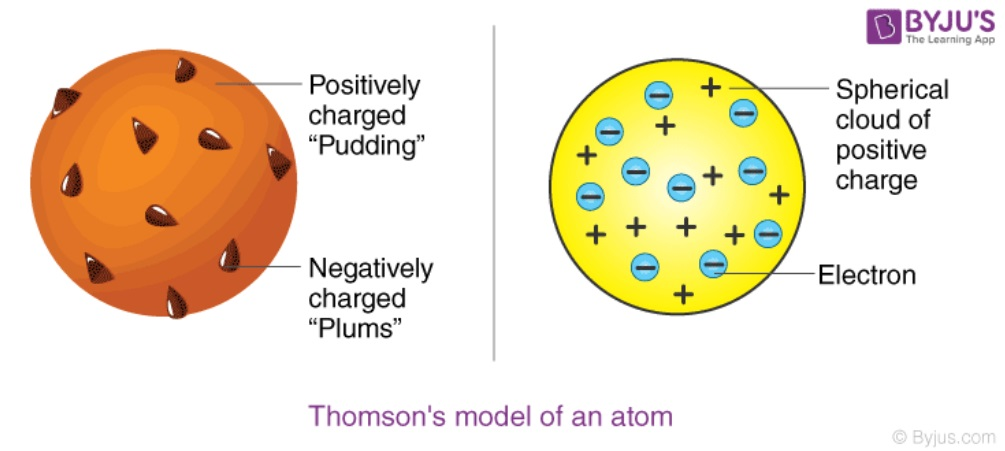7. Rutherford’s Model of Atoms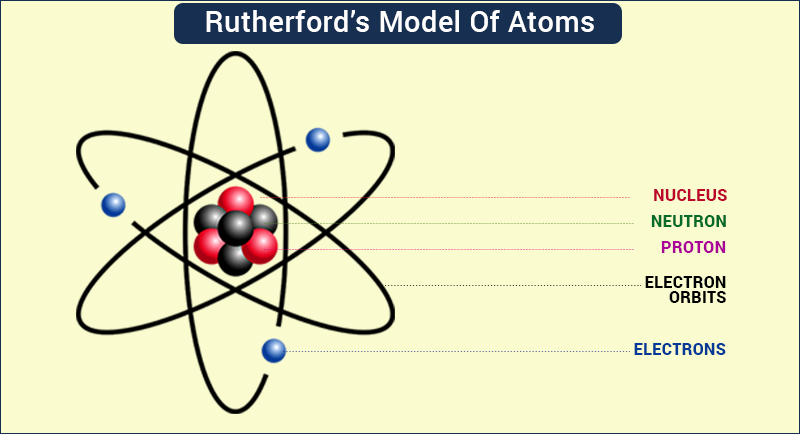8. Bohr’s model of the atomSummarise the rules for writing of distribution of electrons in various shells for the first eighteen elements. Solution:
• Maximum number of electrons that can be accommodated in a shell is given by the formula: 2n2, where n= 1, 2, 3…
• Maximum number of electrons in different shells are:
K shell – n=1 ; 2n2 = 2(1)2 = 2 L shell – n=2 ; 2n2 = 2(2)2 = 8 M shell – n=3 ; 2n2 = 2(3)2 = 18 N shell- n=4 ; 2n2 = 2(4)2 = 32
• The outermost orbit can be accommodated with 8 electrons at the maximum.
• The electrons are not taken in unless the inner shells are filled which are filled step-wise, hence the highest element has K-2; L-8 ; M-8 distribution of electrons.
9. Define valency by taking examples of silicon and oxygen. Solution: The definite combining capacity of the atoms of each element, wherein electrons are lost, gained or shared to make the octet of electrons present in the outermost shell is defined as valency. To measure valency, we can figure out the number of electrons that are required to complete the shell in which it is contained or losing excess electrons if present, once the filling is complete. Example : To find the valency of silicon: The atomic number of silicon is 14 Number of electrons is equal to the number of protons in silicon i.e., 14 The distribution of electrons in silicon atom is K - 2, L - 8, M - 4 Hence, from the distribution of silicon it is clearly evident that to fill the M shell 4 electrons are required. Therefore its valency is 8-4=4. To find the valency of oxygen: The atomic number of oxygen is 8 Number of electrons is equal to the number of protons in oxygen i.e., 8 The distribution of electrons in oxygen atom is K - 2, L - 6 Hence, from the distribution of oxygen it is clearly evident that to fill the M shell 6 more electrons are required. Therefore its valency is 8-6=2. 10. Explain with examples (i) Atomic number, (ii) Mass number, (iii) Isotopes and (iv) Isobars. Give any two uses of isotopes. Solution: (i) The number of positively charged protons present in the nucleus of an atom is defined as the atomic number and is denoted by Z. Example: Hydrogen has one proton in its nucleus, hence its atomic number is one. (ii) The total number of protons and neutrons present in the nucleus of an atom is known as the mass number. It is denoted by A. 20Ca40 . Mass number is 40. Atomic number is 20. (iii) The atoms which have the same number of protons but different number of neutrons are referred to as isotopes. Hence the mass number varies. Example: The most simple example is the Carbon molecule which exists as 6C12 and 6C14 (iv) Isobars: Isobars are atoms which have the same mass number but differ in the atomic number. Examples are,  20Ca40and  18Ar40 Uses of isotopes:
• The isotope of Iodine atom is used to treat goitre and iodine deficient disease.
• In the treatment of cancer, an isotope of cobalt is used.
• Fuel for nuclear reactors is derived from the isotopes of the Uranium atom.
11. Na+ has completely filled K and L shells. Explain. Solution: The atomic number of sodium is 11. It has 11 electrons in its orbitals wherein the number of protons is equal to the number of electrons. Hence, its electronic configuration is K-2 ; L-8 ; M-1 ; The one electron in the M shell is lost and it obtains a positive charge since it has one more proton than electrons, and obtains a positive charge, Na+ . The new electronic configuration is K-1 ; L-8 which is the filled state. Hence it is very difficult to eliminate the electron from a filled state as it is very stable. 12. If bromine atom is available in the form of, say, two isotopes  35Br79 (49.7%) and 35Br81 (50.3%), calculate the average atomic mass of Bromine atom. Solution: The atomic mass of an element is the mass of one atom of that element. Average atomic mass takes into account the isotopic abundance. Isotope of bromine with atomic mass 79 u = 49.7% Therefore, Contribution of 35Br79 to atomic mass = (79 × 49.7)/100 ⇒ 39.26 u Isotope of bromine with atomic mass 81 u = 50.3% Contribution of 35Br81 to the atomic mass of bromine = (81 × 50.3)/100 ⇒ 40.64u Hence, average atomic mass of the bromine atom = 39.26 + 40.64 u = 79.9u 13. The average atomic mass of a sample of an element X is 16.2 u. What are the percentages of isotopes  8X16 and 8X18 in the sample? Solution: Let the percentage of 8X16 be ‘a’ and that of 8X18  be ‘100-a’. As per given data, 16.2u = 16 a / 100 + 18 (100-a) /100 1620 = 16a + 1800 - 18a 1620 = 1800 - 2a a = 90% Hence, the percentage of isotope in the sample 8X16  is 90% and that of 8X18 = 100-a = 100- 90=10% 14. If Z=3, what would be the valency of the element? Also, name the element. Solution: Given: Atomic number, Z = 3 The electronic configuration of the element = K-2; L-1, hence its valency = 1 The element with atomic number 3 is Lithium. 15. Composition of the nuclei of two atomic species X and Y are given as under                           X Y Protons = 6 6 Neutrons = 6 8 Give the mass numbers of X and Y. What is the relation between the two species? Solution: Mass number of X: Protons + neutrons = 6+6 = 12 Mass number of Y: Protons + neutrons = 6+8 = 14 They are the same element as their atomic numbers are the same. They are isotopes as they differ in the number of neutrons and hence their mass numbers. 16. For the following statements, write T for true and F for false. (a) J.J. Thomson proposed that the nucleus of an atom contains only nucleons. (b) A neutron is formed by an electron and a proton combining together. Therefore it is neutral. (c) The mass of an electron is about 1/2000 times that of proton. (d) An isotope of iodine is used for making tincture iodine, which is used as a medicine. Solution: (a) Statement is False (b)  Statement is False (c)  Statement is True (d)  Statement is False 17. Put a tick(✓) against correct choice and cross(x) against wrong choice in questions 15, 16 and 17. Rutherford’s alpha – particle scattering experiment was responsible for the discovery of (a) Atomic nucleus (b) Electron (c) Proton (d) Neutron Solution: (a) Atomic nucleus Isotopes of an element have (a) The same physical properties (b) Different chemical properties (c) Different number of neutrons (d) Different atomic numbers. Solution: (c) Different number of neutrons 18. Number of valence electrons in Cl ion are: (a) 16 (b) 8 (c) 17 (d) 18 Solution: (b) 8 Electronic distribution of Cl is K-2, L-8, M-7. Valence electrons are 7, hence chlorine gains one electron for the formation of Cl-. Therefore, its valency is 8. 19. Which one of the following is a correct electronic configuration of Sodium? (a) 2, 8 (b) 8, 2, 1 (c) 2, 1, 8 (d) 2, 8, 1 Solution: (d) 2, 8, 1 Complete the following table.
 Atomic number Mass number Number of neutrons Number of Protons Number of electrons Name of the atomic species 9 16 – – – – 32 24 2 1 10 – – – 0 – – 12 1 1 – – – – 0 – Sulphur – – –
Solution: The following table depicts the missing data: Atomic number(Z) =Number of protons Mass number = Number of neutrons + atomic number (or) Mass number(A) = Number of neutrons + number of neutrons
 Atomic number Mass number Number of neutrons Number of Protons Number of electrons Name of the atomic species 9 16 12 1 1 19 32 24 2 1 10 16 12 1 0 9 16 12 1 1 9 16 12 1 0 Fluorine Sulphur Magnesium Deuterium Hydrogen

## NCERT Solutions for Class 9 Science Chapter 4 - Structure Of The Atom

Chapter 4 Structure of the atom of NCERT Class 9 Science is categorized under Unit I - Matter - Its nature and behaviour which fundamentally deals with the physical nature of matter and what constitutes the particles that make up matter. The whole unit of Matter makes up for 23 marks out of which the Structure of the atom chapter is allocated 10 marks. In order to score maximum marks, students should practise using the Science NCERT Solutions for Class 9.

This chapter has a good weightage. Thorough knowledge of all the concepts covered in this chapter will help you achieve up to 10 marks from this chapter alone. Around 2 questions with 5 marks each appear in the CBSE Term II examination from this chapter and are expected to appear in the following year too.

### List of subtopics covered in Class 9 Science Chapter 4 Structure Of The Atom:

 Section Topic/Subtopic 4.1 Charged particles in matter 4.2 The structure of an atom 4.2.1 Thomson's model of an atom 4.2.2 Rutherford's model of an atom 4.2.3 Bohr's model of an atom 4.2.4 Neutrons 4.3 How are electrons distributed in different orbits(shells)? 4.4 Valency 4.5 Atomic number and mass number 4.5.1 Atomic number 4.5.2 Mass number 4.6 Isotopes 4.6.1 Isobars
Students can best utilise the NCERT Solutions for Class 9 Science Chapter 4 to make any quick references to comprehend the above and other complex topics with ease. Further, these solutions can be viewed both online and offline as a downloadable PDF.

### List of Exercises in Class 9 Science Chapter 4

Number 4.1 - Charged particles in matter 2 Question ( 2 short) Number 4.2 - The structure of an atom 4 Question ( 4 short) Number 4.2.4 - Neutrons 2 Question ( 2 short) Number 4.3 - How are electrons distributed indifferent orbits(shells)? 2 Question ( 1 long, 1 short) Number 4.4 - Valency 1 Question ( 1 long) Number 4.5 - Atomic number and mass number 2 Question ( 2 long) Number 4.6 - Isotopes 2 Question ( 1 long, 1 short) Exercise Solutions - 19 Question ( 6 long, 9 short, 4 MCQ)

## NCERT Solutions for Class 9 Science Chapter 4 - Structure of the Atom

In this chapter, we get enlightened about the discovery of electrons and protons that is credited to J.J. Thomson and E.Goldstein, respectively. As per the theory by J.J.Thomson, electrons are implanted in the positive sphere. The discovery of the atomic nucleus was forwarded by Rutherford's alpha-particle scattering experiment. We also learn about how Neil Bohr's model was successful as he explained how electrons are distributed in different orbits around the nucleus.

This chapter also briefs about the discovery of neutrons in an atom proposed by J.Chadwick, hence the conclusion about the existence of three sub-atomic particles i.e., protons, neutrons, and electrons. It also sheds light upon concepts such as valency, atomic number and mass number, which are essential to identify elements and their behavioural properties. Learn more about these concepts by accessing the NCERT Solutions for Class 9 of this chapter of Science.

Overall, it helps us answer the basic concepts of what sets an atom apart from atoms of other elements, the indivisibility concept of atoms, representation of elements through mass number and atomic mass, as well as the basic constituents of an atom.

### Key Features of NCERT Solutions for Class 9 Science Chapter 4 - Structure of The Atom

• NCERT Solutions enable students to prepare for CBSE Class 9 Term II examination stress-free.
• Calculations and electronic configurations have been explained in detail while solving.
• Diagrams have been used wherever necessary to be able to learn visually.
• Numericals involving finding valency of elements have been worked out in detail.
• Comparison questions involve the use of tables to help learn and understand better.
• Pointers have been used in the solutions to remember at a glance.
[FAQ_SCHEMA_START]
Frequently Asked Questions on NCERT Solutions for Class 9 Science Chapter 4
List the topics and subtopics covered in Chapter 4 of NCERT Solutions for Class 9 Science.
The topics and subtopics covered in Chapter 4 of NCERT Solutions for Class 9 Science are - 4.1 Charged particles in matter 4.2 The structure of an atom 4.2.1 Thomson’s model of an atom 4.2.2 Rutherford’s model of an atom 4.2.3 Bohr’s model of an atom 4.2.4 Neutrons 4.3 How are electrons distributed in different orbits(shells)? 4.4 Valency 4.5 Atomic number and mass number 4.5.1 Atomic number 4.5.2 Mass number 4.6 Isotopes 4.6.1 Isobars
How many exercises are present in Chapter 4 of NCERT Solutions for Class 9 Science?
The exercises present in Chapter 4 of NCERT Solutions for Class 9 Science are - Number 4.1 – Charged particles in matter 2 Questions ( 2 short) Number 4.2 – The structure of an atom 4 Questions ( 4 short) Number 4.2.4 – Neutrons 2 Questions ( 2 short) Number 4.3 – How are electrons distributed Indifferent orbits(shells) - 2 Questions ( 1 long, 1 short) Number 4.4 – Valency 1 Question ( 1 long) Number 4.5 – Atomic number and mass number 2 Questions ( 2 long) Number 4.6 – Isotopes 2 Questions ( 1 long, 1 short) Exercise Solutions – 19 Questions ( 6 long, 9 short, 4 MCQ)
Where can I find the NCERT Solutions for Class 9 Science Chapter 4 online?
You can find the NCERT Solutions for Class 9 Science Chapter 4 from BYJU’S. The experts have prepared these solutions to help students with their CBSE Term II exam preparation. The solutions contain explanations in understandable language for better conceptual knowledge among students. By using the solutions PDF, students can save a lot of time in finding the correct answer for the respective question.
[FAQ_SCHEMA_END]

#### 1 Comment

1. Byju,s is so good